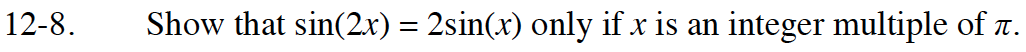Home > CCA2 > Chapter 12 > Lesson 12.1.1 > Problem12-8

12-8.

Show that sin(2x) = 2sin(x) only if x is an integer multiple of π. Use the Desmos calculator below to graph the equation. 12-8 HW eTool (Desmos). Homework Help ✎Graph the equations y = sin (2x) and y = 2 · sin(x) on graphing paper or use Desmos calculator.
Where are the two equations equal?

Use the Desmos calculator below to graph the equations.
Click the link at right for the full version of the eTool: CCA2 12-8 HW eTool.